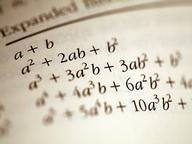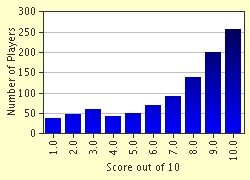FREE! Click here to Join FunTrivia. Thousands of games, quizzes, and lots more!# My Good Friend Slope Trivia Quiz

### In this quiz, my friend slope needs help. Who's better to help him than you? Are you up to the challenge?

A multiple-choice quiz by specialkarah. Estimated time: 4 mins.

Author
specialkarah
Time
4 mins
Type
Multiple Choice
Quiz #
184,515
Updated
Dec 03 21
# Qns
10
Difficulty
Average
Avg Score
7 / 10
Plays
5509
Last 3 plays: briarwoodrose (6/10), AdamM7 (8/10), buckeye52 (2/10).
This quiz has 2 formats: you can play it as a or as shown below.
Scroll down to the bottom for the answer key.
1. First, Slope needs to know what letter is used to represent him in a linear equation. Hint

x
m
b
y

#### NEXT>

2. Slope needs to know which of these equations is in slope-intercept form. Hint

2x - y + 6 = 0
x + y = -1
y = -x + 2
y - 4 = 3x

#### NEXT>

3. Uh-oh! Slope has lost himself in an equation! Can you help me find him? The equation is y = 2x + 5. Hint

5
5/2
1
2

#### NEXT>

4. Oh no! Slope had no idea that there was an equation in his path, and he lost himself yet again! This time, the equation is 1/2x + 2y = 3. Hint

2
1/2
3/2
-1/4

#### NEXT>

5. Slope got lost in yet another equation! Unfortunately, he didn't give me the equation. All he said was that it was parallel to the equation 3x - y = 7. Can you find him? Hint

-3
-1/3
1/3
3

#### NEXT>

6. Slope got lost in yet another equation! Unfortunately, he didn't give me the equation. This time Slope is in an equation perpendicular to the line he gave me. Here it is: 4x + 3y = 9. Hint

3/4
3
-4/3
-1/3

#### NEXT>

7. Now, what is my good friend Slope up to? I can't seem to find him, though I did find a tattered piece of paper with two points on it, (-1,2) and (4,5). Do you think you can find him? Hint

7/3
3
1/3
3/5

#### NEXT>

8. This time, Slope is not lost. He is -1. He still needs your help though. Can you help him find the equation he is in that runs through the point (0,6)? Hint

y = -6x + 1
y = 6x - 1
y = -x + 6
y = x - 6

#### NEXT>

9. Slope is 1/2, but he needs an equation of a line that passes through the point (-2,-3). Use him to find one. Hint

x - 2y = 4
x + 2y = -4
x + 1/2 y = 1/4
x - 1/2 y = -1/4

#### NEXT>

10. Slope has stumbled across a weird equation. Your final test is to find him in it. The peculiar equation is x = 3. Hint

1
undefined
0
3

 (Optional) Create a Free FunTrivia ID to save the points you are about to earn:Select a User ID:Choose a Password:Your Email:

Most Recent Scores
Sep 24 2023 : briarwoodrose: 6/10
Sep 23 2023 : AdamM7: 8/10
Sep 14 2023 : buckeye52: 2/10
Sep 13 2023 : Guest 165: 3/10
Sep 09 2023 : orugtor: 9/10
Sep 01 2023 : Guest 208: 7/10
Aug 25 2023 : Guest 94: 3/10

Score DistributionQuiz Answer Key and Fun Facts
1. First, Slope needs to know what letter is used to represent him in a linear equation.

Slope thanks you if you got this correct! How is he supposed to do anything if he doesn't know that he is represented by an "m" in a linear equation? The "b" is the y-intercept, which is where the line crosses the y-axis on a graph. "x" and "y" are just variables; the "y" depends on what you plug in for "x" and, therefore, is known as the dependent variable.
2. Slope needs to know which of these equations is in slope-intercept form.

Answer: y = -x + 2

Slope feels ashamed for not knowing this! When his memory came back, he told me to tell you that this is correct because it is in "y = mx + b" form. x + y = -1 is not an accurate answer because it is in "standard form". In order to change y - 4 = 3x to slope-intercept form, you have to add four to both sides. Slope-intercept form for the equation 2x - y + 6 = 0 is obtained by doing this:
2x - y + 6 = 0
-2x -2x
- y + 6 = -2x
- 6 - 6
-y = -2x - 6
Divide each term by a -1.
y = 2x + 6
3. Uh-oh! Slope has lost himself in an equation! Can you help me find him? The equation is y = 2x + 5.

Slope thanks you again for helping him get out of that jam! For those who are ignorant of Algebra, Slope wants you to know that whenever a linear equation is in slope-intercept form, whatever number is in front of the independent variable "x" is the slope, or "m".
4. Oh no! Slope had no idea that there was an equation in his path, and he lost himself yet again! This time, the equation is 1/2x + 2y = 3.

Whew! In order to find Slope in this equation, you must first put the equation in standard form. You may ask: isn't it already in standard form? The answer Slope told me to give you is that the coefficent (number multiplied "x" times) must be a whole number before an equation is in standard form. To make 1/2 a whole number, you must multiply through the whole equation by two. Now the equation is x + 4y = 6. There are now two ways to obtain the number for slope. The first is to subtract x from both sides, like so:
x + 4y = 6
-x -x
4y = 6 - x
Now, divide all terms by 4 change the equation to slope-intercept form.
y = -1/4x + 3/2
Therefore, Slope is -1/4 in this equation.
Another name for standard form is Ax + By = C form.
To obtain the slope for an equation while it is in standard form, use the formula -A/B.
In our equation, A is 1 and B is 4
-A/B = -1/4
Once again, Slope is -1/4
5. Slope got lost in yet another equation! Unfortunately, he didn't give me the equation. All he said was that it was parallel to the equation 3x - y = 7. Can you find him?

Parallel means same slope. Therefore, the equation Slope is in has the same slope as the given equation. To find the slope of 3x - y = 7, first subtract 3x from both sides.
3x - y = 7
-3x -3x
-y = 7 - 3x
Divide all terms by a -1 and switch the term with the variable around with the term lacking a variable to put in slope-intercept form.
y = 3x - 7
The slope is 3.
Slope is in an equation with the same slope as this one.
Slope is 3.
6. Slope got lost in yet another equation! Unfortunately, he didn't give me the equation. This time Slope is in an equation perpendicular to the line he gave me. Here it is: 4x + 3y = 9.

Perpendicular means to take the opposite reciprocal of the slope of a line. First, let's put the equation in slope-intercept form:
4x + 3y = 9
-4x -4x
3y = -4x + 9
Divide all terms by 3.
y = -4/3x + 3
The slope is -4/3.
Slope is in an equation that is perpendicular to this one.
The opposite of -4/3 is 4/3.
The reciprocal of that is 3/4.
Slope is 3/4.
7. Now, what is my good friend Slope up to? I can't seem to find him, though I did find a tattered piece of paper with two points on it, (-1,2) and (4,5). Do you think you can find him?

The slope formula for finding slope is "y sub two minus y sub one over x sub two minus x sub one." It does not matter which point you use for (x,y) one or (x,y) two. In this case, I'll use -1 as "x sub one" and 2 as "y sub one."
"Y sub two" is 5, and "x sub two" is four.
5 - 2 = 3
4 - (-1) = 4 + 1
4 + 1 = 5
Slope is 3/5.
8. This time, Slope is not lost. He is -1. He still needs your help though. Can you help him find the equation he is in that runs through the point (0,6)?

Answer: y = -x + 6

As I've mentioned before, "b" is the y-intercept. The y-intercept is where the equation of the line crosses the y-axis and x is 0. Therefore, the point (0,6) is the y-intercept. For this problem, all you had to do was slip Slope in for "m" and the y-intercept in for "b" to arrive at your answer.
9. Slope is 1/2, but he needs an equation of a line that passes through the point (-2,-3). Use him to find one.

Answer: x - 2y = 4

y = mx + b
y = -3, x = -2, m = 1/2, b is unknown.
-3 = 1/2(-2) + b
-3 = -1 + b
+1 +1
-2 = b
Final equation: y = 1/2x - 2
This is not an answer choice.
All answer choices are in standard form.
Let's change our equation to a standard form one.
y = 1/2x - 2
-1/2x -1/2x
-1/2x + y = -2
Make the coefficient in front of x a positive whole number.
-2(-1/2x + y = -2)
x - 2y = 4
Alas, we have our equation.
To check, plug the point in for x and y.
(-2) -2(-3) = 4
-2 + 6 = 4
4 = 4
The answer is most definitely x - 2y = 4.
10. Slope has stumbled across a weird equation. Your final test is to find him in it. The peculiar equation is x = 3.

This equation would be a vertical line on a graph. Slope is undefined because when you take two points on the line, say (3,1) and (3,3), and plug the numbers in the slope formula, you get 2/0. 2 cannot divide by 0 since nothing times 0 is ever going to give you 2, and therefore it is undefined. If the equation had been y = 3, Slope would have been 0. Plug (2,3) and (8,3) in the slope formula and you will get 0/6. 0 times 6 = 0 and in this case Slope is 0. Thanks so much for helping Slope out today! If you learned something from this quiz, don't hesitate to send me a message. So long for now!
Source: Author specialkarah

This quiz was reviewed by FunTrivia editor crisw before going online.
Any errors found in FunTrivia content are routinely corrected through our feedback system.
Related Quizzes
1. The Matrix Average
2. An Adventure in Abstract Algebra Very Difficult
3. Basic Math or Algebra? Tough
4. Algebra Wonderland! Tough